## sklearn实战-----9.sklearn中的线性回归大家族

2020/7/24 11:26:30 文章标签: 测试文章如有侵权请发送至邮箱809451989@qq.com投诉后文章立即删除

# 1 概述

## 1.2 sklearn中的线性回归

sklearn中的线性模型模块是linear_model，我们曾经在学习逻辑回归的时候提到过这个模块。linear_model包含了多种多样的类和函数，其中逻辑回归相关的类和函数在这里就不给大家列举了。今天的课中我将会为大家来讲解：普通线性回归，多项式回归，岭回归，LASSO，以及弹性网。

linear_model.LinearRegression 使用普通最小二乘法的线性回归

linear_model.Ridge 岭回归，一种将L2作为正则化工具的线性最小二乘回归
linear_model.RidgeCV 带交叉验证的岭回归
linear_model.RidgeClassifier 岭回归的分类器
linear_model.RidgeClassifierCV 带交叉验证的岭回归的分类器
linear_model.ridge_regression 【函数】用正太方程法求解岭回归
LASSO
linear_model.Lasso Lasso，使用L1作为正则化工具来训练的线性回归模型
linear_model.LassoCV 带交叉验证和正则化迭代路径的Lasso
linear_model.LassoLars 使用最小角度回归求解的Lasso
linear_model.LassoLarsCV 带交叉验证的使用最小角度回归求解的Lasso
linear_model.LassoLarsIC 使用BIC或AIC进行模型选择的，使用最小角度回归求解的Lasso
linear_model.lasso_path 【函数】用坐标下降计算Lasso路径

linear_model.ElasticNet 弹性网，一种将L1和L2组合作为正则化工具的线性回归
linear_model.ElasticNetCV 带交叉验证和正则化迭代路径的弹性网
linear_model.enet_path 【函数】用坐标下降法计算弹性网的路径

linear_model.Lars 最小角度回归（Least Angle Regression，LAR）
linear_model.LarsCV 带交叉验证的最小角度回归模型
linear_model.lars_path 【函数】使用LARS算法计算最小角度回归路径或Lasso的路径

linear_model.OrthogonalMatchingPursuit 正交匹配追踪模型（OMP）
linear_model.OrthogonalMatchingPursuitCV 交叉验证的正交匹配追踪模型（OMP）
linear_model.orthogonal_mp 【函数】正交匹配追踪（OMP）
linear_model.orthogonal_mp_gram 【函数】Gram正交匹配追踪（OMP）

linear_model.ARDRegression 贝叶斯ARD回归。ARD是自动相关性确定回归（Automatic Relevance Determination Regression），是一种类似于最小二乘的，用来计算参数向量的数学方法。
linear_model.BayesianRidge 贝叶斯岭回归

linear_model.PassiveAggressiveClassifier 被动攻击性分类器
linear_model.PassiveAggressiveRegressor 被动攻击性回归
linear_model.Perceptron 感知机
linear_model.RANSACRegressor RANSAC（RANdom SAmple Consensus）算法。
linear_model.HuberRegressor 胡博回归，对异常值具有鲁棒性的一种线性回归模型
linear_model.SGDRegressor 通过最小化SGD的正则化损失函数来拟合线性模型
linear_model.TheilSenRegressor Theil-Sen估计器，一种鲁棒的多元回归模型

# 2 多元线性回归LinearRegression

## 2.1 多元线性回归的基本原理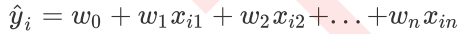$w$被统称为模型的参数，其中$w_0$被称为截距(intercept)，$w_1$~$w_n$ 被称为回归系数(regression coefficient)，有时也是使用$\theta$或者$\beta$来表示。这个表达式，其实就和我们小学时就无比熟悉的$y=ax+b$是同样的性质。其中$y$是我们的目标变量，也就是标签。 $x_{i1}$~$x_{in}$ 是样本$i$上的特征不同特征。如果考虑我们有m个样本，则回归结果可以被写作：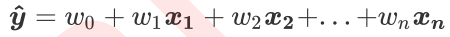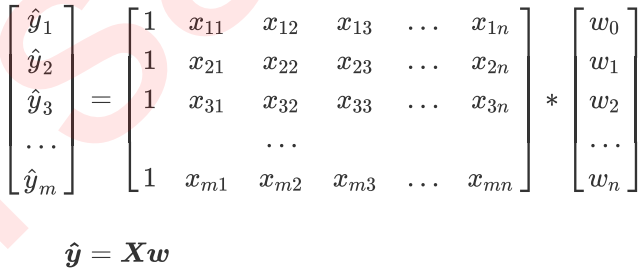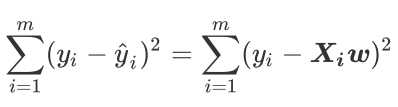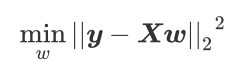## 2.2 最小二乘法求解多元线性回归的参数

https://en.wikipedia.org/wiki/Matrix_calculus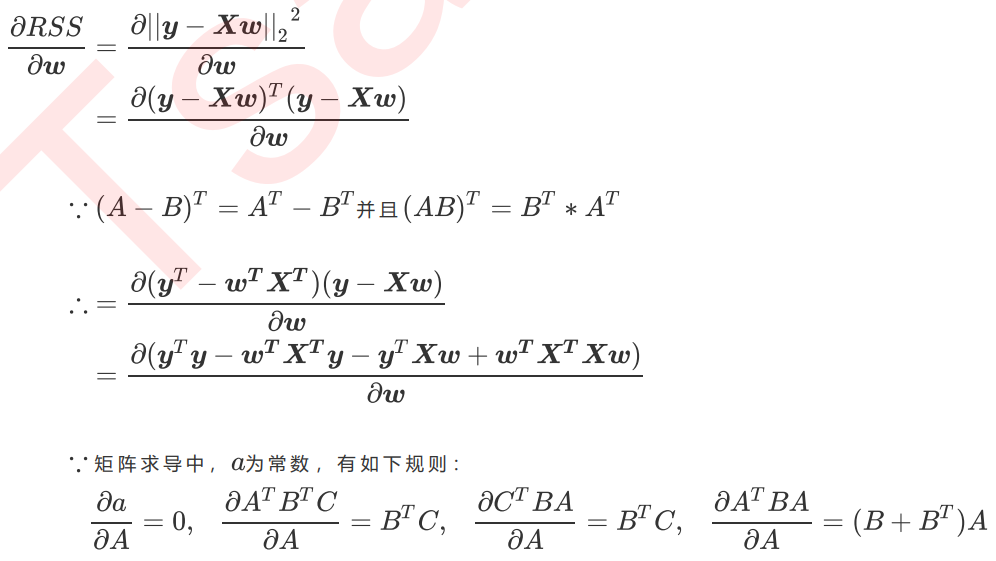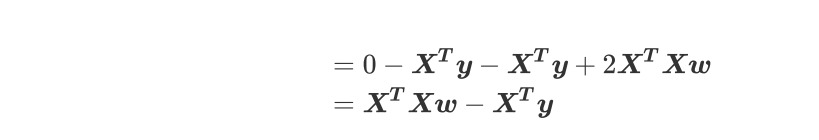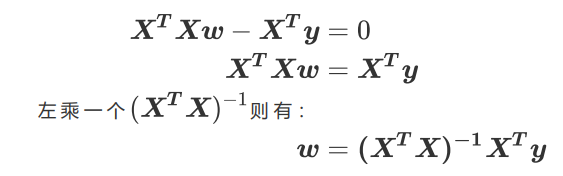## 2.3 linear_model.LinearRegression

class sklearn.linear_model.LinearRegression (fit_intercept=True,
normalize=False, copy_X=True, n_jobs=None)

fit_intercept 布尔值，可不填，默认为True；是否计算此模型的截距。如果设置为False，则不会计算截距
normalize 布尔值，可不填，默认为False；当fit_intercept设置为False时，将忽略此参数。如果为True，则特征矩阵X在进入回归之前将；会被减去均值（中心化）并除以L2范式（缩放）。如果你希望进行标准化，请在fit数据之前；使用preprocessing模块中的标准化专用类StandardScaler
copy_X 布尔值，可不填，默认为True；如果为真，将在X.copy()上进行操作，否则的话原本的特征矩阵X可能被线性回归影响并覆盖
n_jobs 整数或者None，可不填，默认为None；用于计算的作业数。只在多标签的回归和数据量足够大的时候才生效。除非None在joblib.parallel_backend上下文中，否则None统一表示为1。如果输入 -1，则表示使用全部的CPU来进行计算。

• 来做一次回归试试看吧
1. 导入需要的模块和库
from sklearn.linear_model import LinearRegression as LR
from sklearn.model_selection import train_test_split
from sklearn.model_selection import cross_val_score
from sklearn.datasets import fetch_california_housing as fch #加利福尼亚房屋价值数据集
import pandas as pd

1. 导入数据，探索数据
housevalue = fch() #会需要下载，大家可以提前运行试试看

housevalue.data

X = pd.DataFrame(housevalue.data) #放入DataFrame中便于查看

X.shape
#(20640, 8)

y = housevalue.target
y.min()
#0.14999
y.max()
#5.00001
y.shape
#(20640,)

housevalue.feature_names #特征名字

X.columns = housevalue.feature_names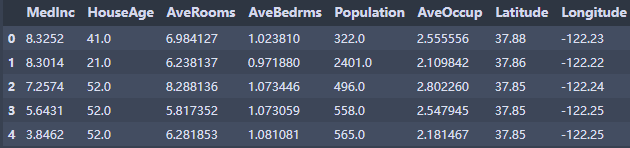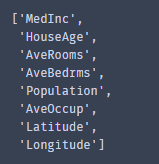"""
MedInc：该街区住户的收入中位数
HouseAge：该街区房屋使用年代的中位数
AveRooms：该街区平均的房间数目
AveBedrms：该街区平均的卧室数目
Population：街区人口
AveOccup：平均入住率
Latitude：街区的纬度
Longitude：街区的经度
"""

1. 分训练集和测试集
Xtrain, Xtest, Ytrain, Ytest = train_test_split(X,y,test_size=0.3,random_state=420)

#恢复索引
for i in [Xtrain, Xtest]:
i.index = range(i.shape)

Xtrain.shape
# (14448, 8)

#如果希望进行数据标准化，还记得应该怎么做吗？
#先用训练集训练(fit)标准化的类，然后用训练好的类分别转化(transform)训练集和测试集

1. 建模
reg = LR().fit(Xtrain, Ytrain)
yhat = reg.predict(Xtest) #预测我们的yhat
yhat.min()
#-0.6528439725035966
yhat.max()
#7.146198214270875

1. 探索建好的模型
reg.coef_ #w,系数向量

[*zip(Xtrain.columns,reg.coef_)]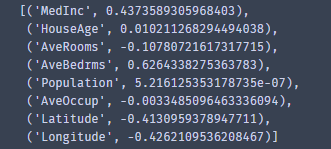"

""
MedInc：该街区住户的收入中位数
HouseAge：该街区房屋使用年代的中位数
AveRooms：该街区平均的房间数目
AveBedrms：该街区平均的卧室数目
Population：街区人口
AveOccup：平均入住率
Latitude：街区的纬度
Longitude：街区的经度
"""

reg.intercept_
#-36.25689322920386


coef_ 数组，形状为 (n_features, )或者(n_targets, n_features)；线性回归方程中估计出的系数。如果在fit中传递多个标签（当y为二维或以上的时候），则返回的系数是形状为（n_targets，n_features）的二维数组，而如果仅传递一个标签，则返回的系数是长度为n_features的一维数组
intercept_ 数组，线性回归中的截距项。

# 3 回归类的模型评估指标

## 3.1 是否预测了正确的数值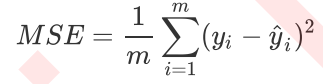from sklearn.metrics import mean_squared_error as MSE
MSE(yhat,Ytest)
#0.5309012639324571
Ytest.mean()
#2.0819292877906976
y.max()
#5.00001
y.min()
#0.14999

cross_val_score(reg,X,y,cv=10,scoring="mean_squared_error")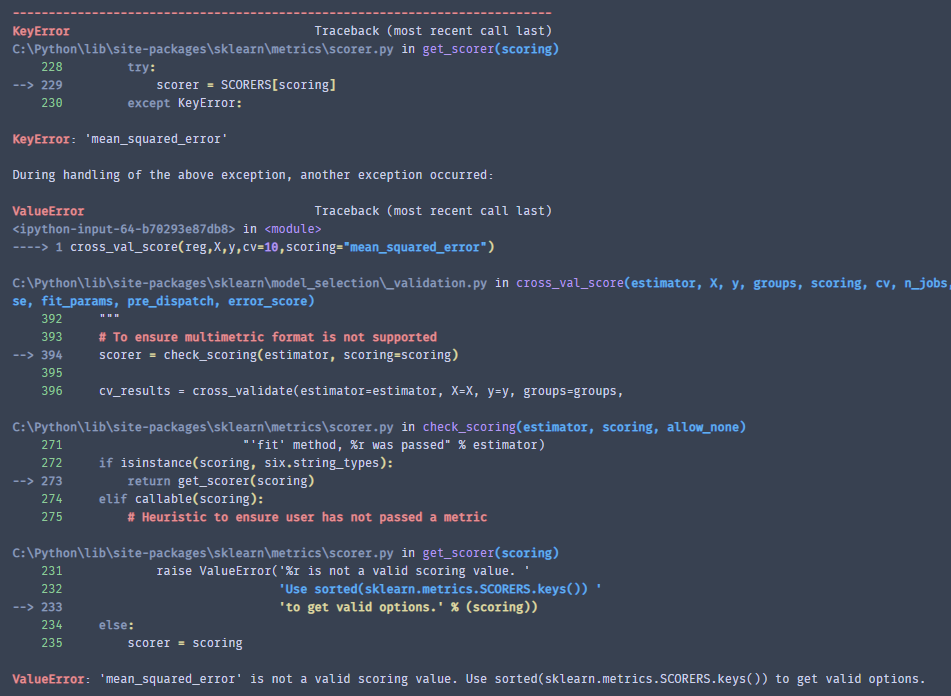#为什么报错了？来试试看！
import sklearn
sorted(sklearn.metrics.SCORERS.keys())
cross_val_score(reg,X,y,cv=10,scoring="neg_mean_squared_error")
# -0.550952429695658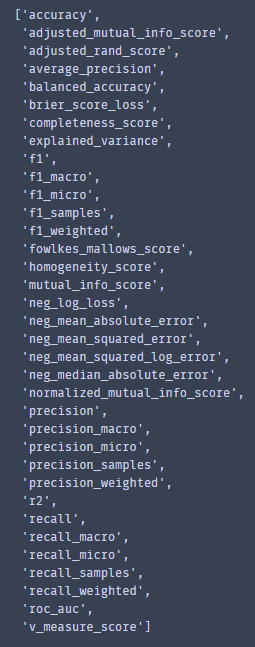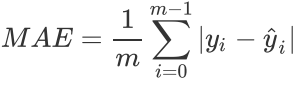## 3.2 是否拟合了足够的信息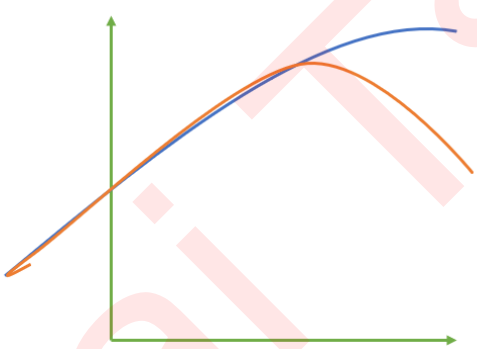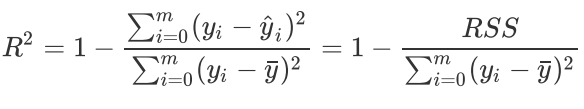$R^2$可以使用三种方式来调用，一种是直接从metrics中导入r2_score，输入预测值和真实值后打分。第二种是直接从线性回归LinearRegression的接口score来进行调用。第三种是在交叉验证中，输入"r2"来调用。

#调用R2
from sklearn.metrics import r2_score
r2_score(yhat,Ytest)
#0.33806537615560006

r2 = reg.score(Xtest,Ytest)
r2
#0.6043668160178817


#使用shift tab键来检查究竟哪个值先进行输入
r2_score(Ytest,yhat)
#0.6043668160178817

#或者你也可以指定参数，就不必在意顺序了
r2_score(y_true = Ytest,y_pred = yhat)
#0.6043668160178817

cross_val_score(reg,X,y,cv=10,scoring="r2").mean()
#0.5110068610524564


import matplotlib.pyplot as plt
sorted(Ytest)

plt.plot(range(len(Ytest)),sorted(Ytest),c="black",label= "Data")
plt.plot(range(len(yhat)),sorted(yhat),c="red",label = "Predict")
plt.legend()
plt.show()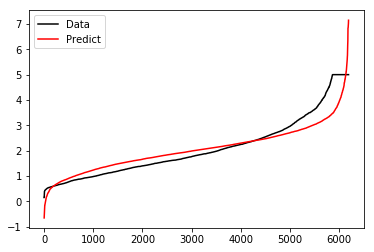import numpy as np
rng = np.random.RandomState(42)
X = rng.randn(100, 80)
y = rng.randn(100)
cross_val_score(LR(), X, y, cv=5, scoring='r2')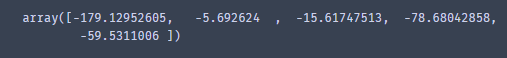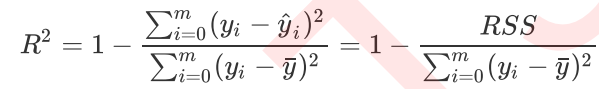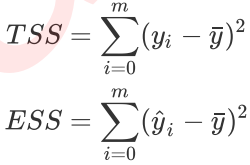$TSS=RSS+ESS$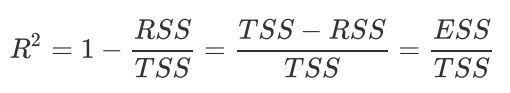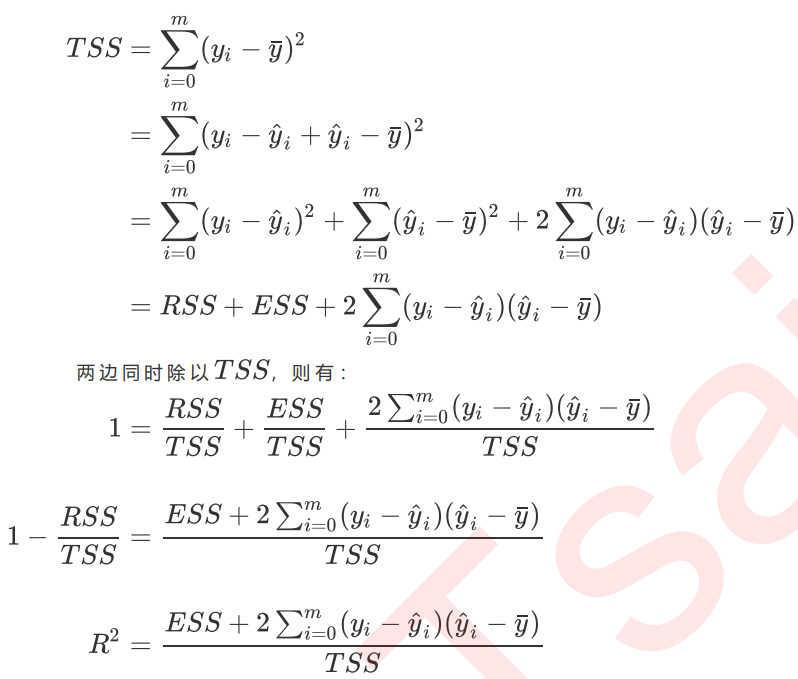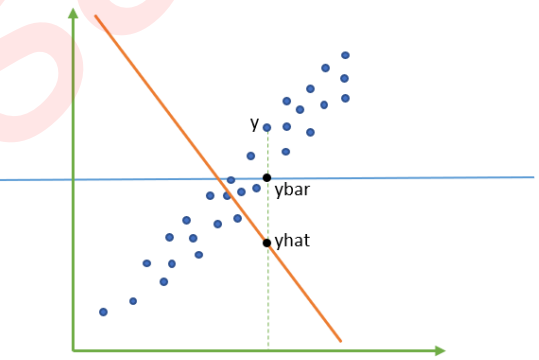# 4 多重共线性：岭回归与Lasso

## 4.1 最熟悉的陌生人：多重共线性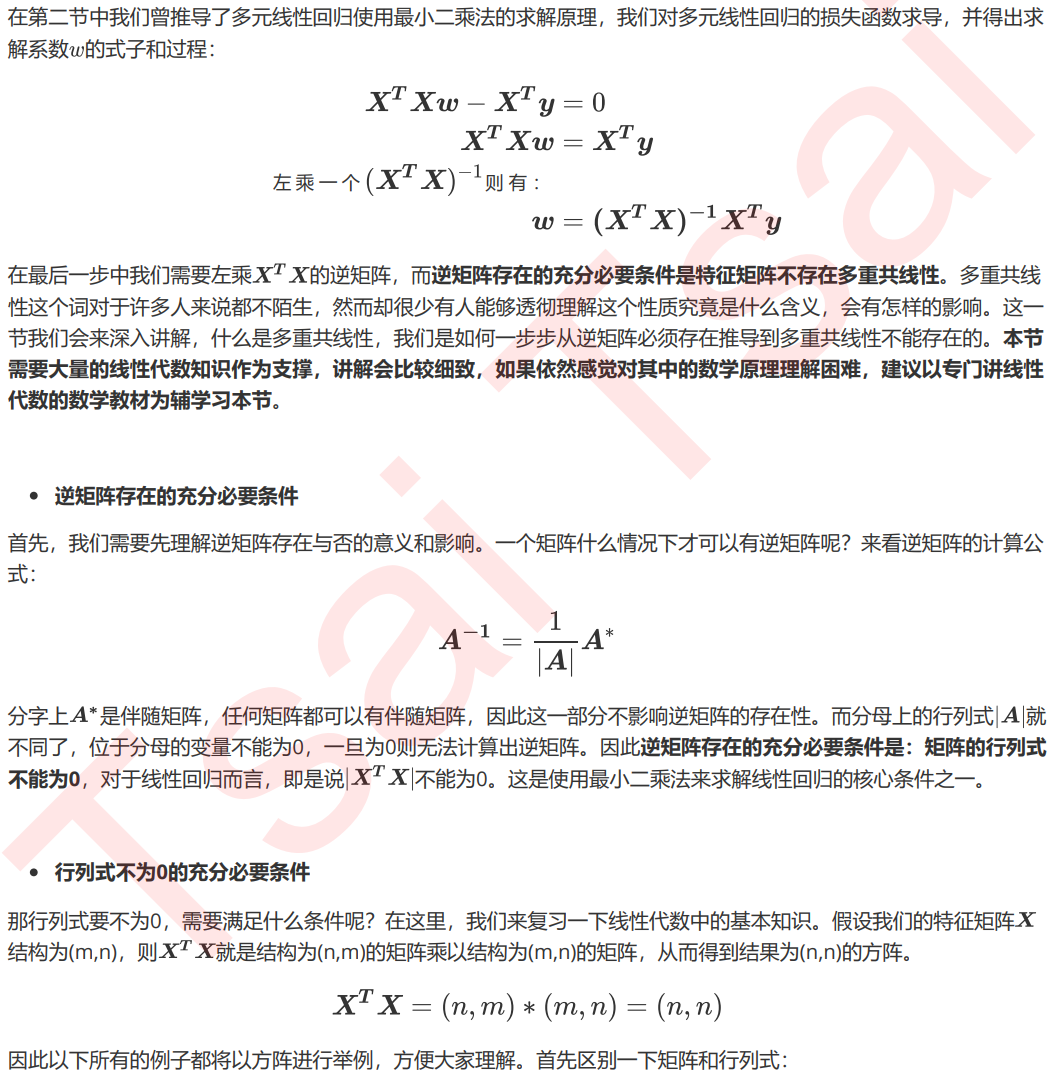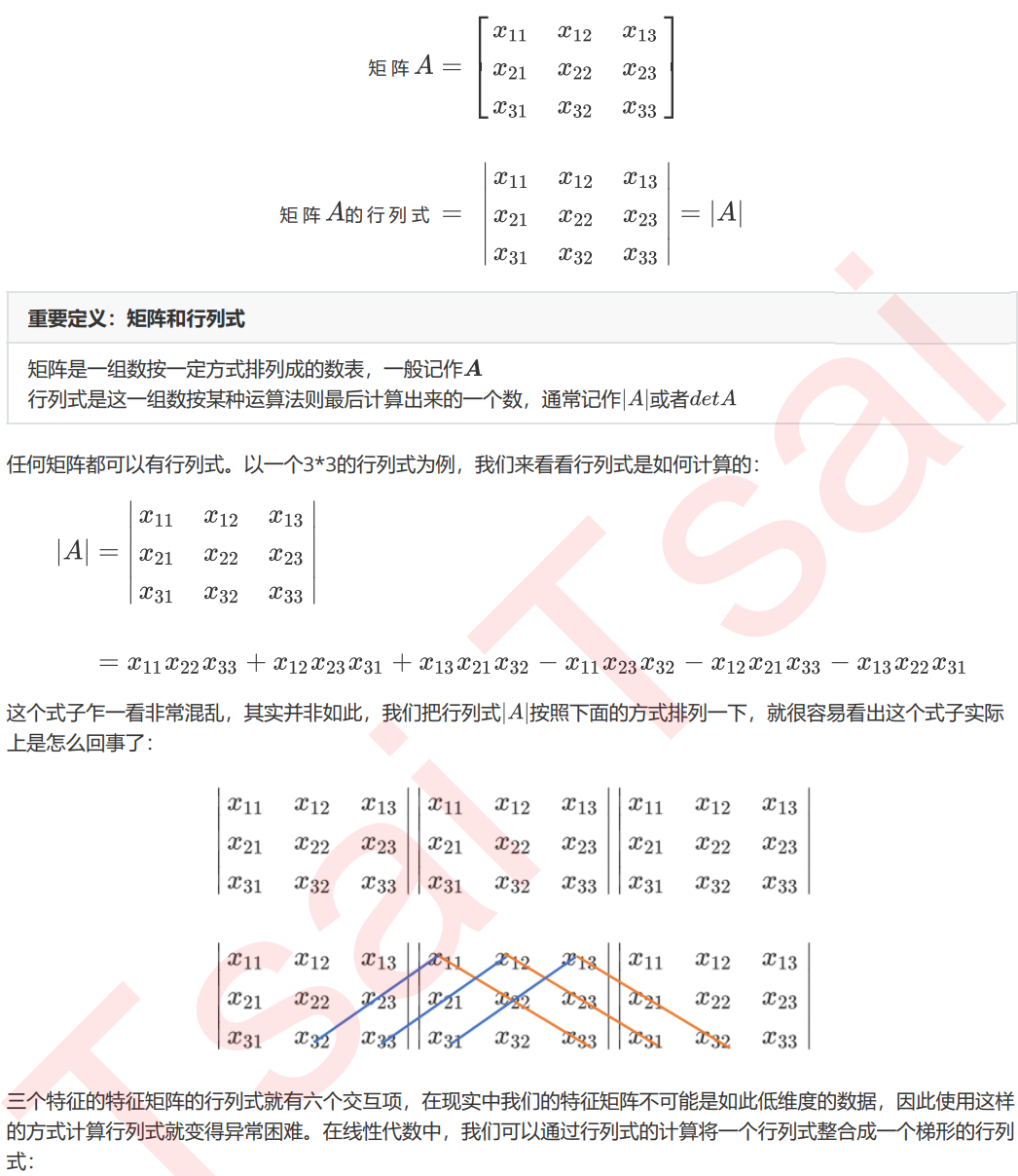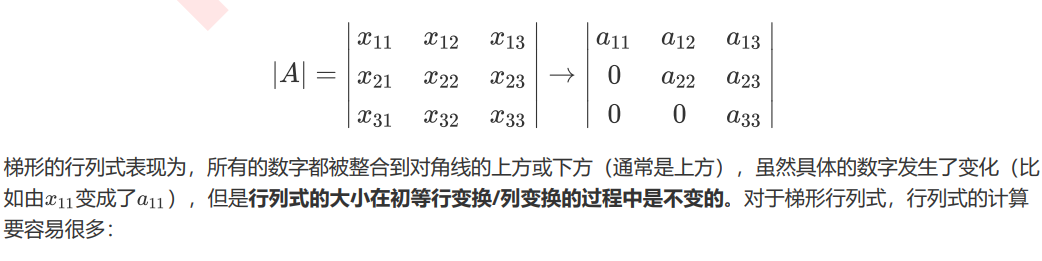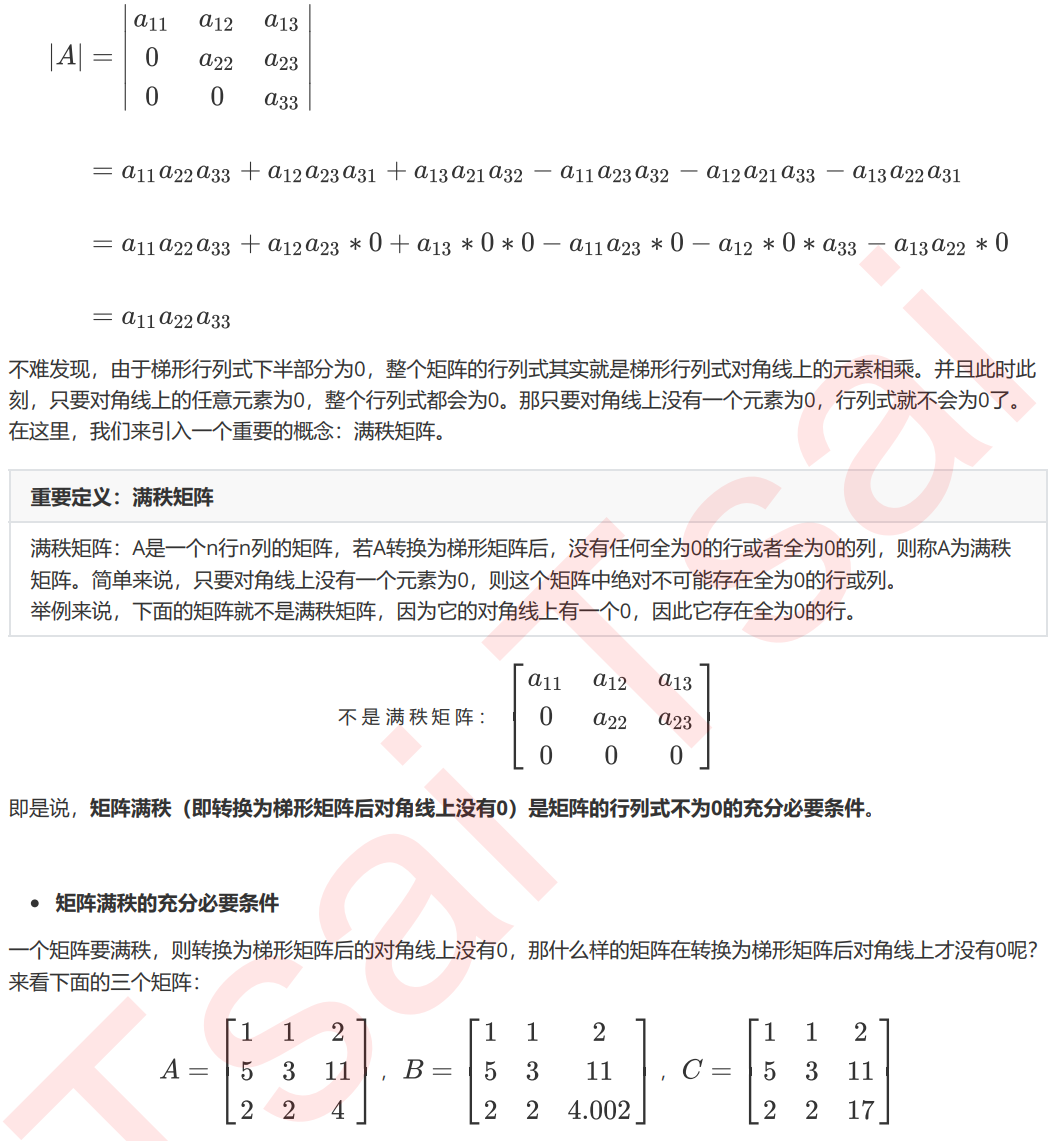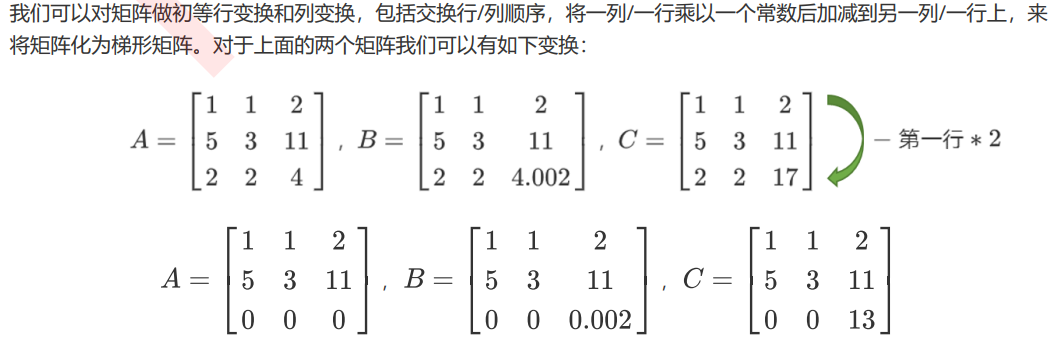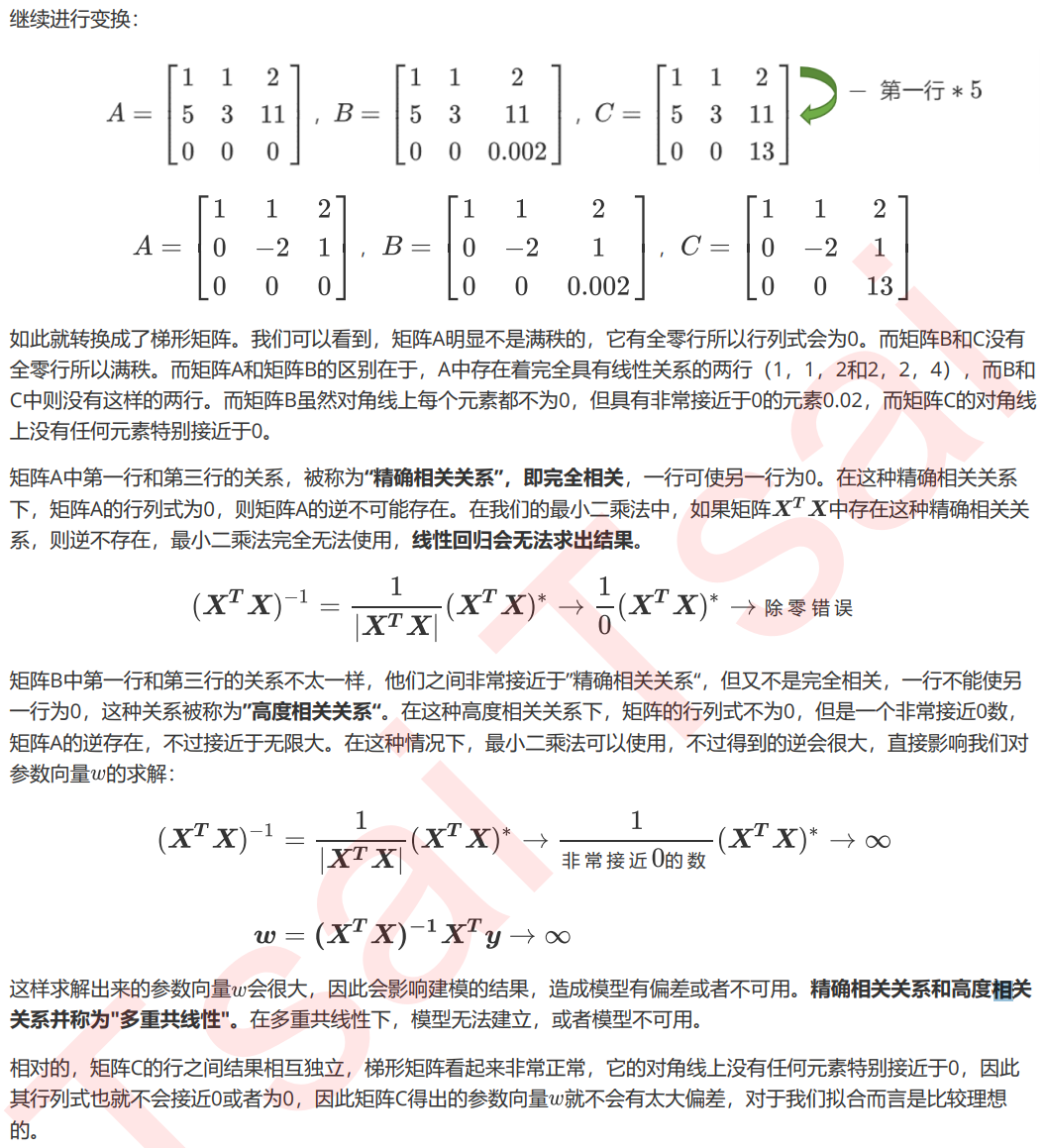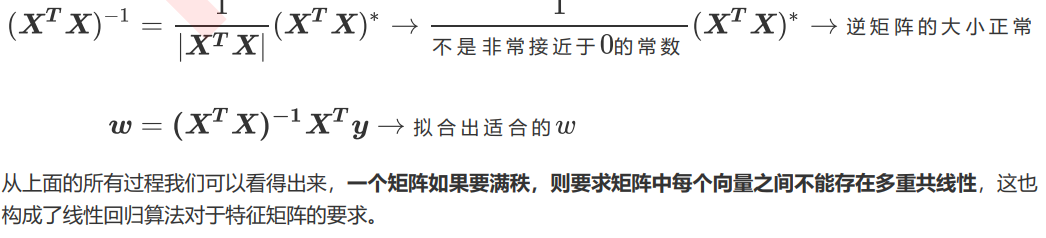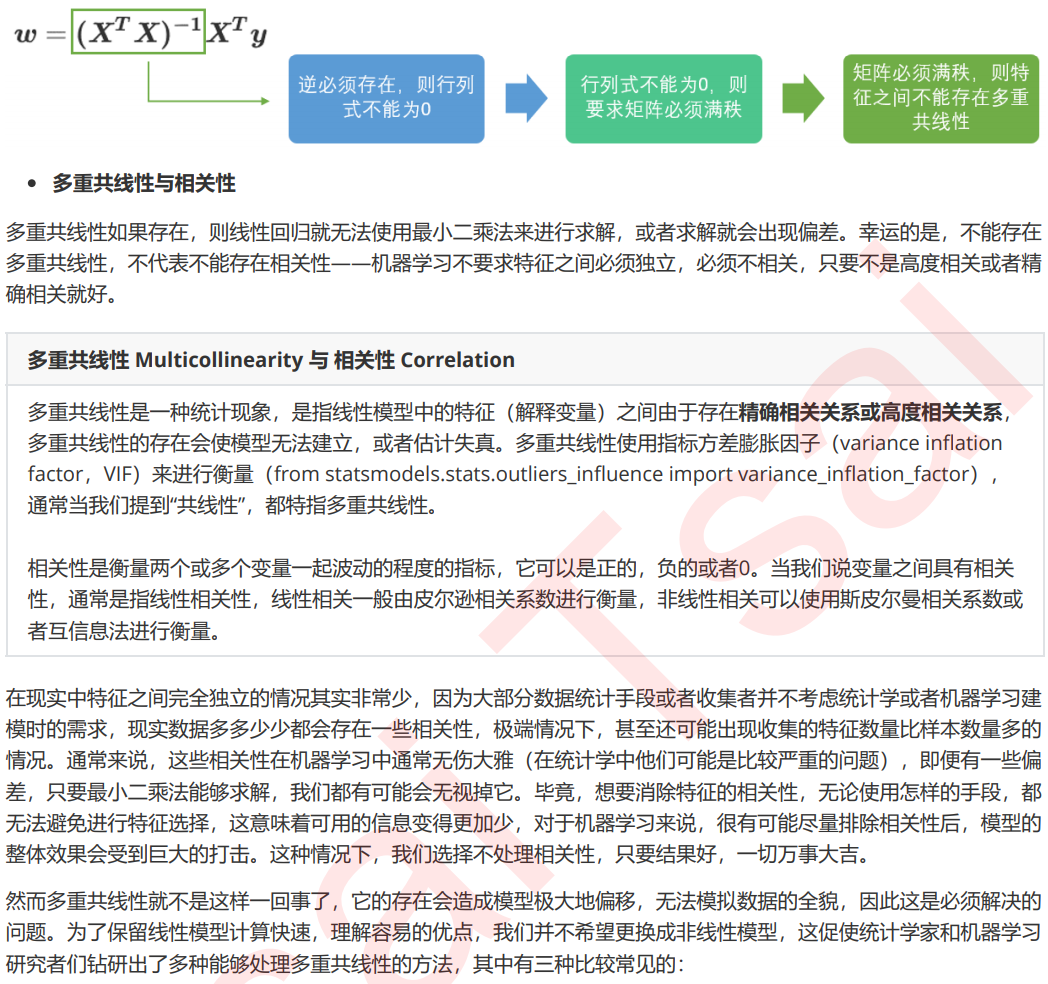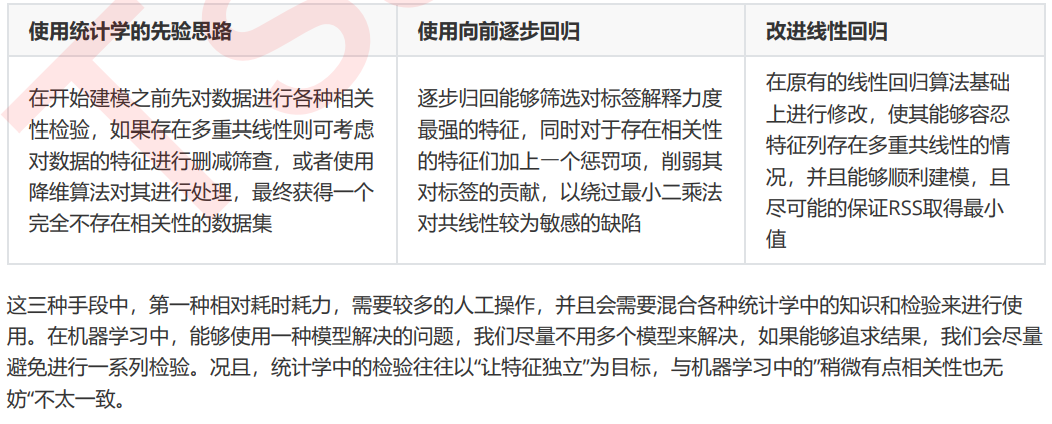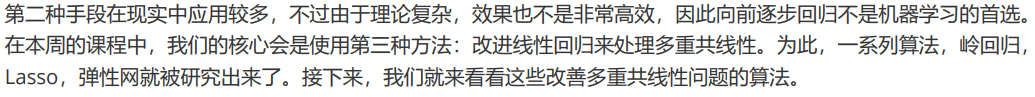## 4.2 岭回归

### 4.2.1 岭回归解决多重共线性问题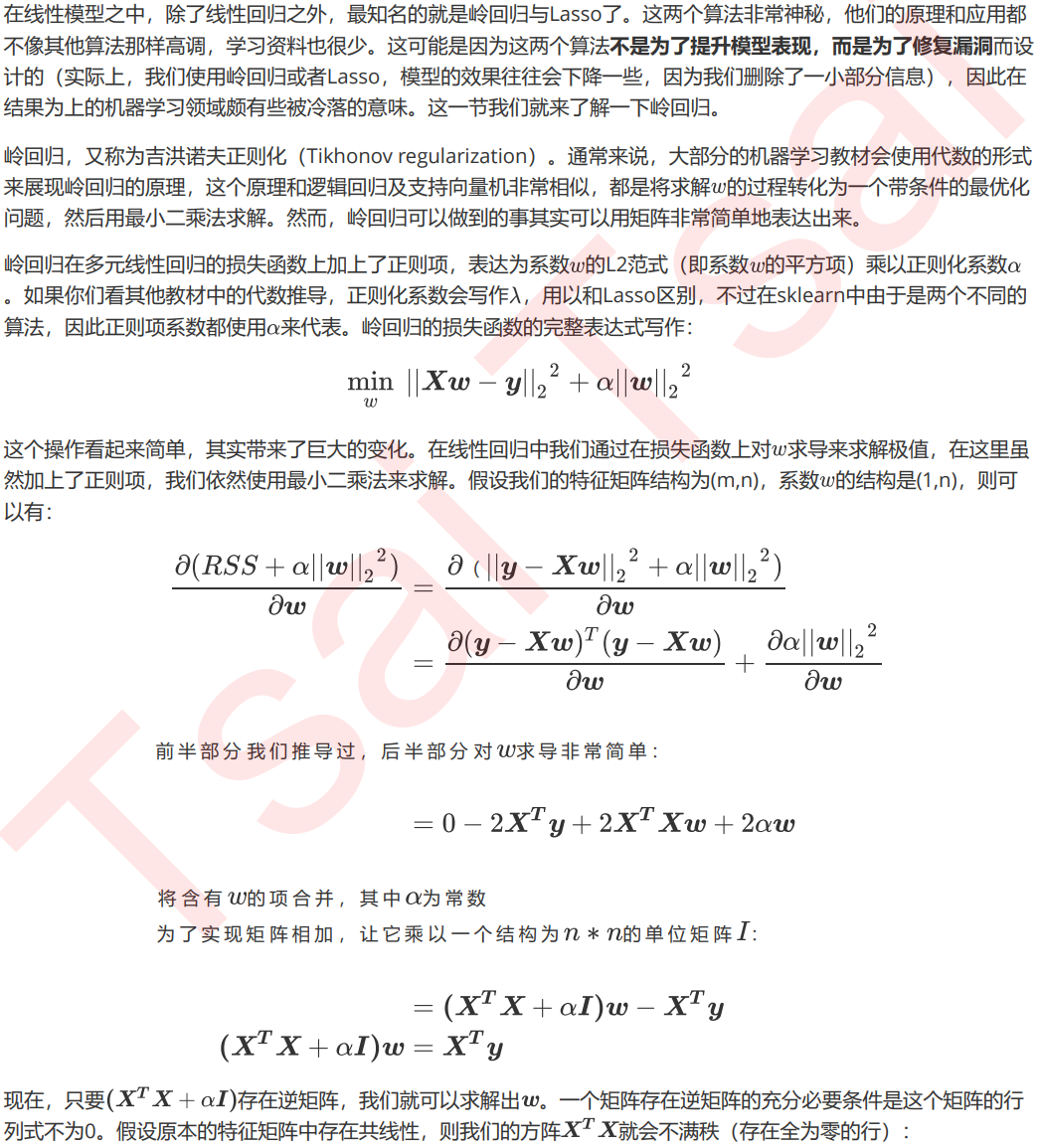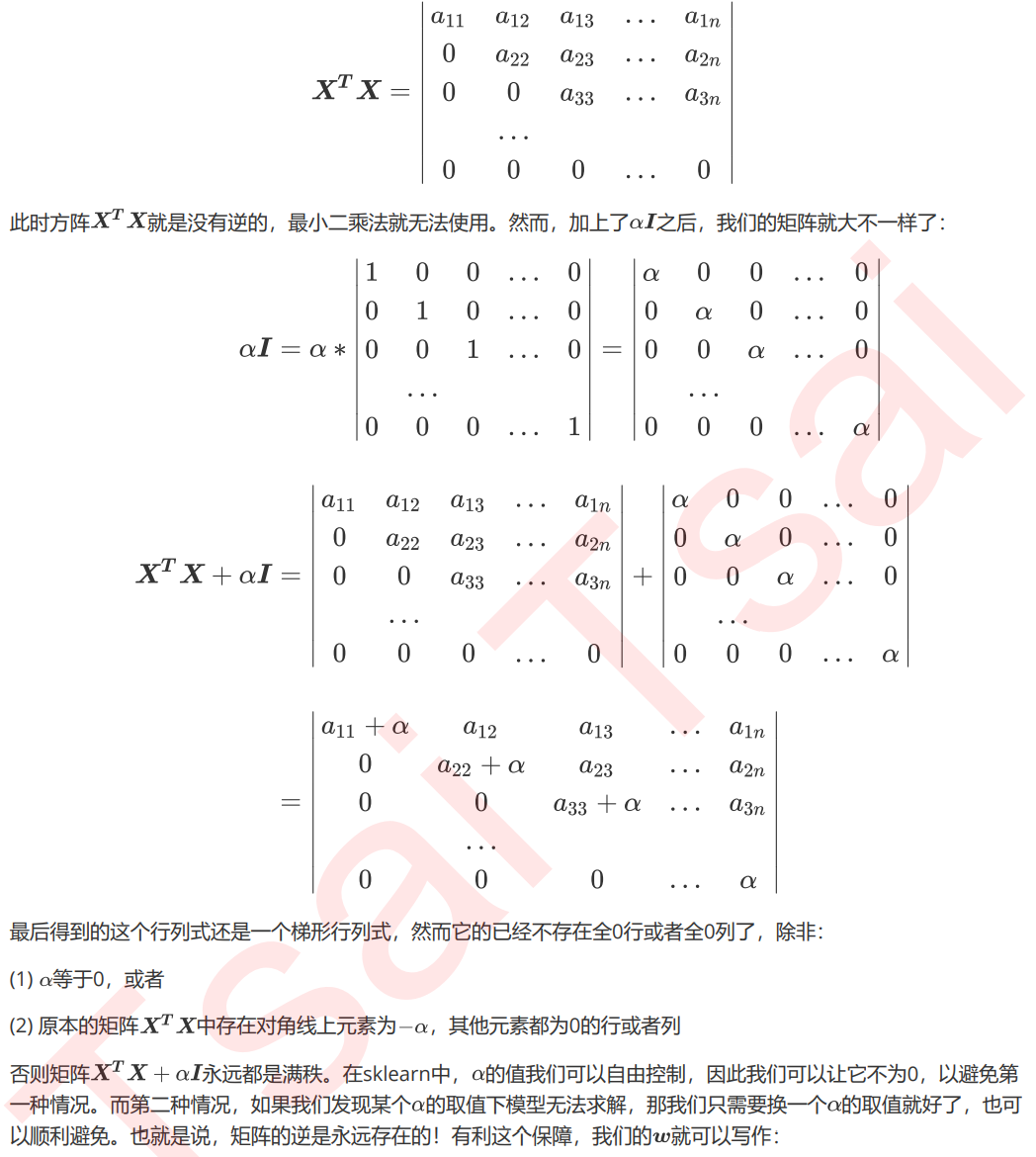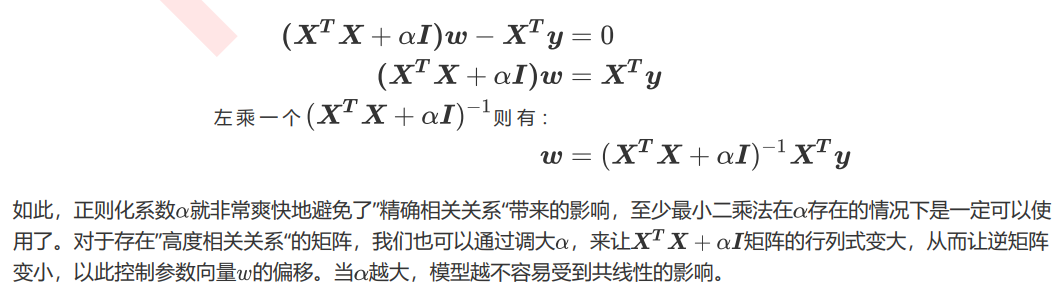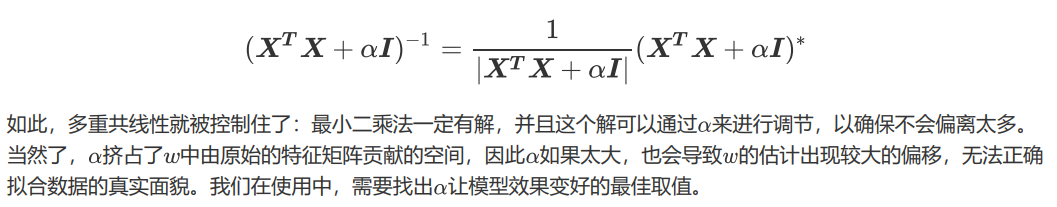### 4.2.2 linear_model.Ridge

class sklearn.linear_model.Ridge (alpha=1.0, fit_intercept=True,
normalize=False, copy_X=True, max_iter=None, tol=0.001, solver=’auto’,
random_state=None)

import numpy as np
import pandas as pd
from sklearn.linear_model import Ridge, LinearRegression, Lasso
from sklearn.model_selection import train_test_split as TTS
from sklearn.datasets import fetch_california_housing as fch
import matplotlib.pyplot as plt

housevalue = fch()

X = pd.DataFrame(housevalue.data)
y = housevalue.target
X.columns = ["住户收入中位数","房屋使用年代中位数","平均房间数目"
,"平均卧室数目","街区人口","平均入住率","街区的纬度","街区的经度"]

Xtrain,Xtest,Ytrain,Ytest = TTS(X,y,test_size=0.3,random_state=420)

#数据集索引恢复
for i in [Xtrain,Xtest]:
i.index = range(i.shape)

#使用岭回归来进行建模
reg = Ridge(alpha=1).fit(Xtrain,Ytrain)

reg.score(Xtest,Ytest)

#交叉验证下，与线性回归相比，岭回归的结果如何变化？
alpharange = np.arange(1,1001,100)
ridge, lr = [], []
for alpha in alpharange:
reg = Ridge(alpha=alpha)
linear = LinearRegression()
regs = cross_val_score(reg,X,y,cv=5,scoring = "r2").mean()
linears = cross_val_score(linear,X,y,cv=5,scoring = "r2").mean()
ridge.append(regs)
lr.append(linears)
plt.plot(alpharange,ridge,color="red",label="Ridge")
plt.plot(alpharange,lr,color="orange",label="LR")
plt.title("Mean")
plt.legend()
plt.show()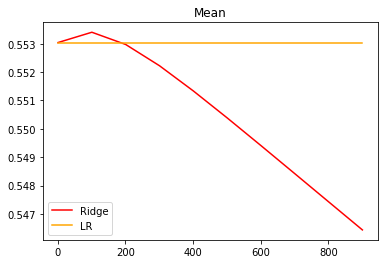#使用岭回归来进行建模
reg = Ridge(alpha=0).fit(Xtrain,Ytrain)
reg.score(Xtest,Ytest) #加利佛尼亚房屋价值数据集中应该不是共线性问题

#细化一下学习曲线
alpharange = np.arange(1,201,10)
ridge, lr = [], []
for alpha in alpharange:
reg = Ridge(alpha=alpha)
linear = LinearRegression()
regs = cross_val_score(reg,X,y,cv=5,scoring = "r2").mean()
linears = cross_val_score(linear,X,y,cv=5,scoring = "r2").mean()
ridge.append(regs)
lr.append(linears)
plt.plot(alpharange,ridge,color="red",label="Ridge")
plt.plot(alpharange,lr,color="orange",label="LR")
plt.title("Mean")
plt.legend()
plt.show()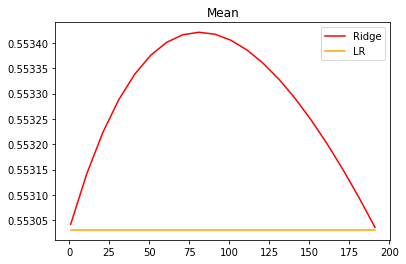#模型方差如何变化？
alpharange = np.arange(1,1001,100)
ridge, lr = [], []
for alpha in alpharange:
reg = Ridge(alpha=alpha)
linear = LinearRegression()
varR = cross_val_score(reg,X,y,cv=5,scoring="r2").var()
varLR = cross_val_score(linear,X,y,cv=5,scoring="r2").var()
ridge.append(varR)
lr.append(varLR)
plt.plot(alpharange,ridge,color="red",label="Ridge")
plt.plot(alpharange,lr,color="orange",label="LR")
plt.title("Variance")
plt.legend()
plt.show()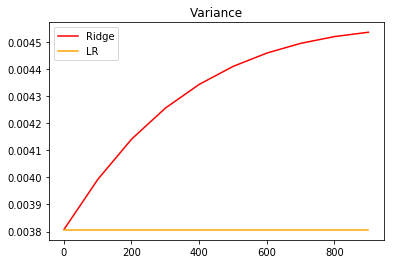from sklearn.datasets import load_boston
from sklearn.model_selection import cross_val_score

Xtrain,Xtest,Ytrain,Ytest = TTS(X,y,test_size=0.3,random_state=420)

X.shape
#(506, 13)

#先查看方差的变化
alpharange = np.arange(1,1001,100)
ridge, lr = [], []
for alpha in alpharange:
reg = Ridge(alpha=alpha)
linear = LinearRegression()
varR = cross_val_score(reg,X,y,cv=5,scoring="r2").var()
varLR = cross_val_score(linear,X,y,cv=5,scoring="r2").var()
ridge.append(varR)
lr.append(varLR)
plt.plot(alpharange,ridge,color="red",label="Ridge")
plt.plot(alpharange,lr,color="orange",label="LR")
plt.title("Variance")
plt.legend()
plt.show()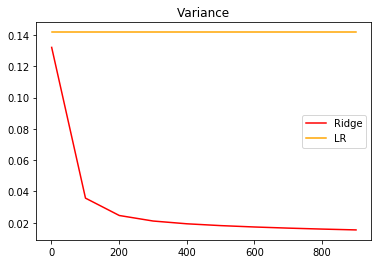#查看R2的变化
alpharange = np.arange(1,1001,100)
ridge, lr = [], []
for alpha in alpharange:
reg = Ridge(alpha=alpha)
linear = LinearRegression()
regs = cross_val_score(reg,X,y,cv=5,scoring = "r2").mean()
linears = cross_val_score(linear,X,y,cv=5,scoring = "r2").mean()
ridge.append(regs)
lr.append(linears)
plt.plot(alpharange,ridge,color="red",label="Ridge")
plt.plot(alpharange,lr,color="orange",label="LR")
plt.title("Mean")
plt.legend()
plt.show()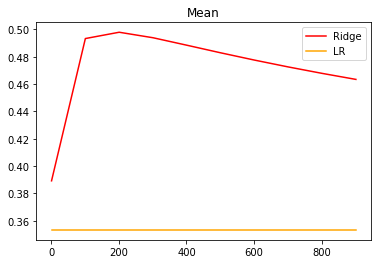#细化学习曲线
alpharange = np.arange(100,300,10)
ridge, lr = [], []
for alpha in alpharange:
reg = Ridge(alpha=alpha)
#linear = LinearRegression()
regs = cross_val_score(reg,X,y,cv=5,scoring = "r2").mean()
#linears = cross_val_score(linear,X,y,cv=5,scoring = "r2").mean()
ridge.append(regs)
lr.append(linears)
plt.plot(alpharange,ridge,color="red",label="Ridge")
#plt.plot(alpharange,lr,color="orange",label="LR")
plt.title("Mean")
plt.legend()
plt.show()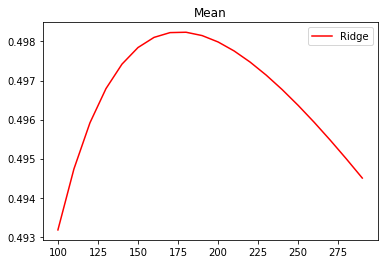可以发现，比起加利佛尼亚房屋价值数据集，波士顿房价数据集的方差降低明显，偏差也降低明显，可见使用岭回归还是起到了一定的作用，模型的泛化能力是有可能会上升的。

### 4.2.3 选取最佳的正则化参数取值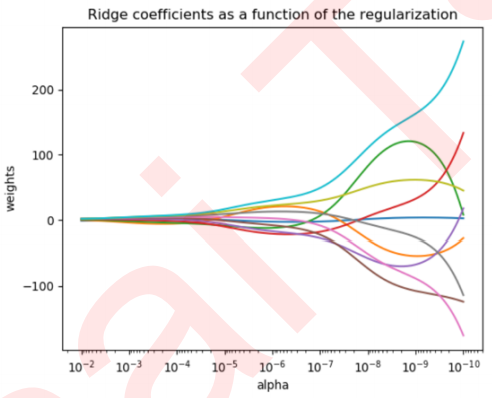暂无相关的数据...